# i dont understand how to find <4,23> Given the following page table for a process, convert...

i dont understand how to find <4,23>

Given the following page table for a process, convert the given logical addresses of the process to the corresponding physical addresses. Page size is 5K bytes.

 Page table 2 10 12 5 8 12 6 13
 Logical address Physical address <4, 23> <1, 2005> <10, 255> <3, 1000> <7, 530> <6, 3000>

 Page Table Base Length 0 219 600 1 2500 11 2 86 160 3 1528 970 4 726 68

23<68

23+726=749

Physical address for <4,23> is 749

##### Add Answer of: i dont understand how to find <4,23> Given the following page table for a process, convert...
Similar Homework Help Questions
• ### 1. Given the following physical addresses and value in memory: add 0 val 9 10 11 12 13 1415161181...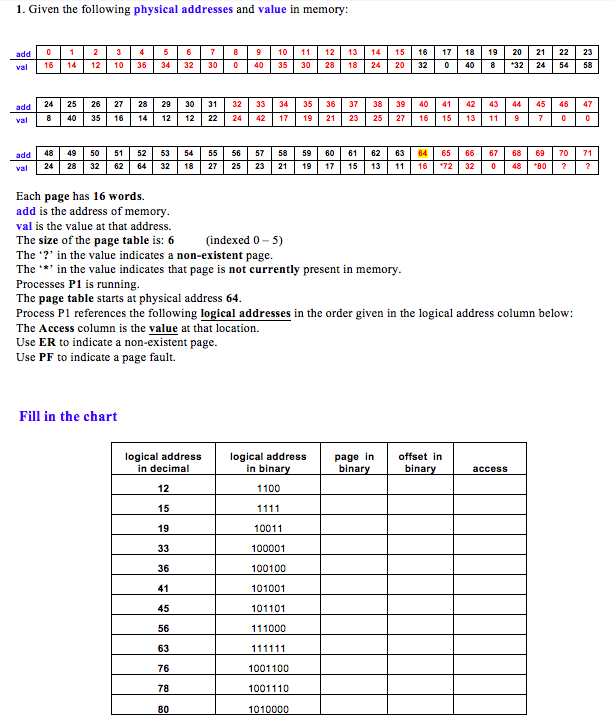1. Given the following physical addresses and value in memory: add 0 val 9 10 11 12 13 14151611819 2021 22 23 18 24 20 32 0 40 8 32 245458 10 36 34 3230 40 35 3028 add 24 25 26 27 28 29 30 31 32 33 34 | 35 | 36 37 38 39 40 | 41 | 42 | 43 44 45 46 47 8 40 35 1614 12 12 22 24417 21 23 25 27...

• ### Problem 6 (13 points) The page table below is for a system with 16-bit virtual as well as physica...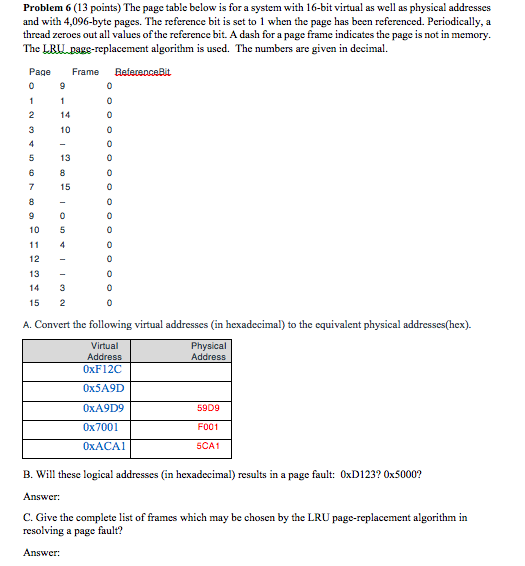Problem 6 (13 points) The page table below is for a system with 16-bit virtual as well as physical addresses and with 4,096-byte pages. The reference bit is set to 1 when the page has been referenced. Periodically, a thread zeroes out all values of the reference bit. A dash for a page frame indicates the page is not in memory. The LRll pagg-replacement algorithm is used. The numbers are given in decimal Page Frame eferepceit 14 10 13 15...

• ### 18. You have a byte-addressable virtual memory system with a two-entry TLB, a 2-way set associati...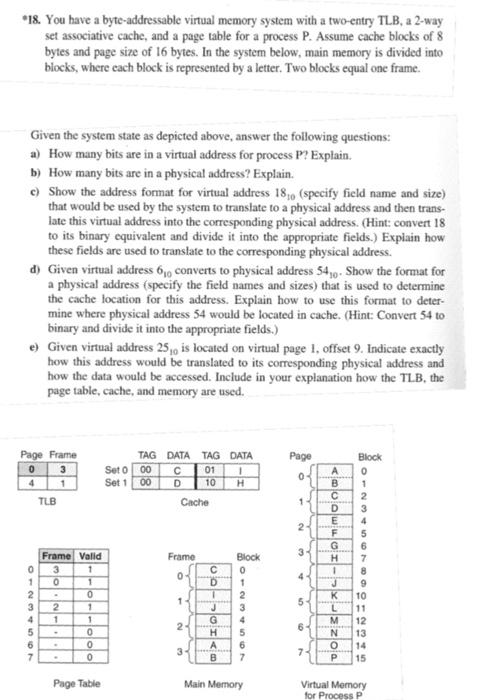18. You have a byte-addressable virtual memory system with a two-entry TLB, a 2-way set associative cache, and a page table for a process P. Assume cache blocks of 8 bytes and page size of 16 bytes. In the system below, main memory is divided into blocks, where each block is represented by a letter. Two blocks equal one frame. Given the system state as depicted above, answer the following questions: a) How many bits are in a virtual address...

• ### 1. Given the following physical addresses and value in memory: Val | 16 | 14 | 12 | 10|36 | 34|32...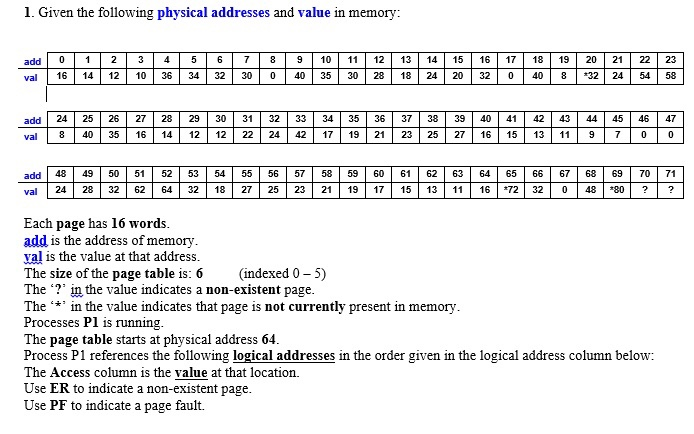1. Given the following physical addresses and value in memory: Val | 16 | 14 | 12 | 10|36 | 34|32 | 30 | 0|40 | 35 | 30 | 28 | 18 | 24 | 20 | 32 | 0|4018|132 | 24 | 54 add 24 25 26 27 28 29 30 31 32 33 34 35 36 37 38 39 40 41 42 4344454647 val 8403516 14121222244217 19 2123252716 1513 119700 add 48 49551 52 53 54 55...

• ### Exercise l: Suppose that we have a virtual memory space of 28 bytes for a given process and physical memory of 4 page frames. There is no cache. Suppose that pages are 32 bytes in length. 1) How...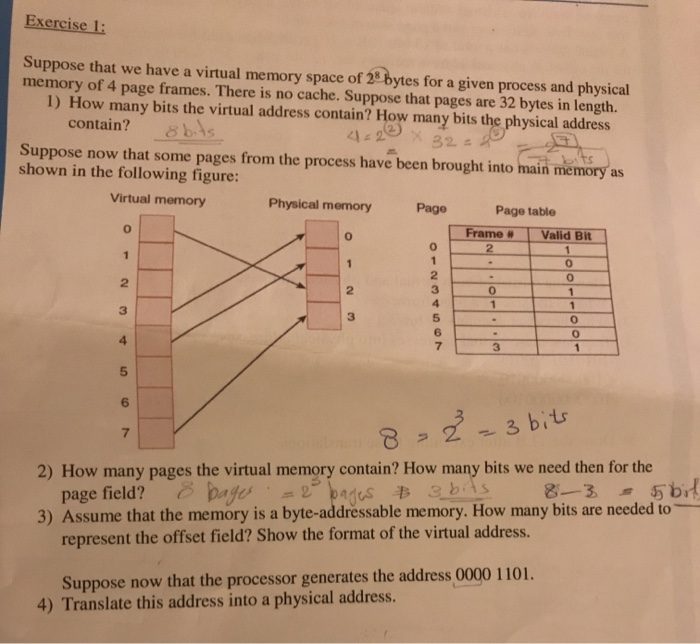Exercise l: Suppose that we have a virtual memory space of 28 bytes for a given process and physical memory of 4 page frames. There is no cache. Suppose that pages are 32 bytes in length. 1) How many bits the virtual address contain? How many bits the physical address contain? bs Suppose now that some pages from the process have been brought into main memory as shown in the following figure: Virtual memory Physical memory Page table Frame #...

• ### Operating System

[Question: 1 Marks: 2*5=10]Suppose that the logical address space of a process consist of 32pages and each page consist of 2 bytes. The main memory consists of32 frames and the size of eachframe is also 2 bytes. Calculate thefollowing from the given information:a) Calculate the size of logical address space of the process?b) Calculate the physical address space of the main memory?c) Calculate the no. of bits required for the Page Number‘p’?d) Calculate the no. of bits required for offset‘d’?e)...

• ### Fill in the table below based on the reference string (RS) shown. The resident set at time 0 is g...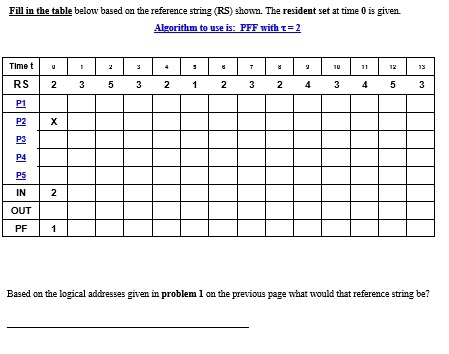Fill in the table below based on the reference string (RS) shown. The resident set at time 0 is given Algorithm to use is: PFF with t-2 RS 2 35 3 212 3 2 4 3 45 3 P1 P2 )X P3 P4 P5 IN 2 OUT PF 1 Based on the logical adáresses given in problem 1 on the previous page what would that reference string be? 1. Given the following physical addresses and value in memory: Val |...

• ### Address Translation Question [8 points] Suppose a computing system uses paging with a logical add...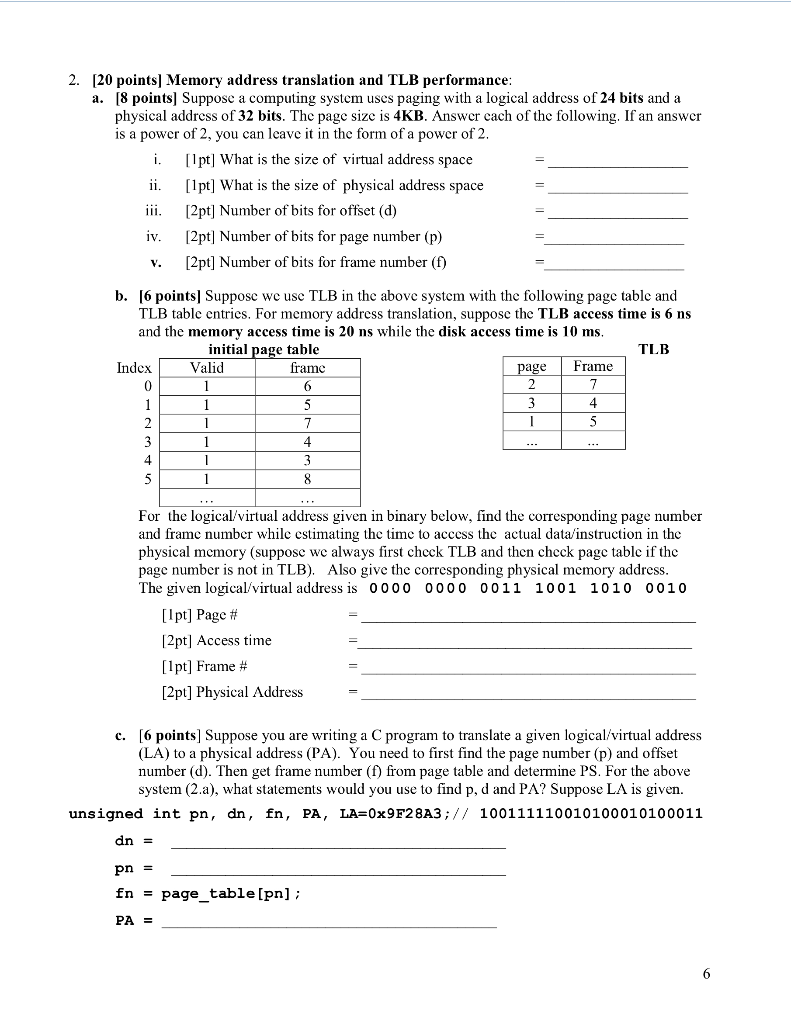Address Translation Question [8 points] Suppose a computing system uses paging with a logical address of 24 bits and a physical address of 32 bits. The page size is 4KB. Answer each of the following. If an answer is a power of 2, you can leave it in the form of a power of 2. ... 2. [20 points] Memory address translation and TLB performance [8 points] Suppose a computing system uses paging with a logical address of 24 bits...

• ### cs604 operating system

[Question: 1Marks: 2*5=10]Suppose that the logical address space of a process consist of32 pages and each page consist of 2 bytes. The main memory consistsof 32 frames and the size of eachframe is also 2 bytes. Calculatethe following from the given information:a)Calculate the size of logicaladdress space of the process?b)Calculate the physical addressspace of the main memory?c)Calculate the no. of bitsrequired for the Page Number ‘p’?d)Calculate the no. of bitsrequired for offset ‘d’?e)Find the number of entries inthe page table?[Question:...

• ### 3) Memory Management [24 pts 12 extra credit] Consider the following segment table: Base 255 400 1924 50 3313 1150 2B90 125 4871 What are the physical addresses for the following logical addresses fo...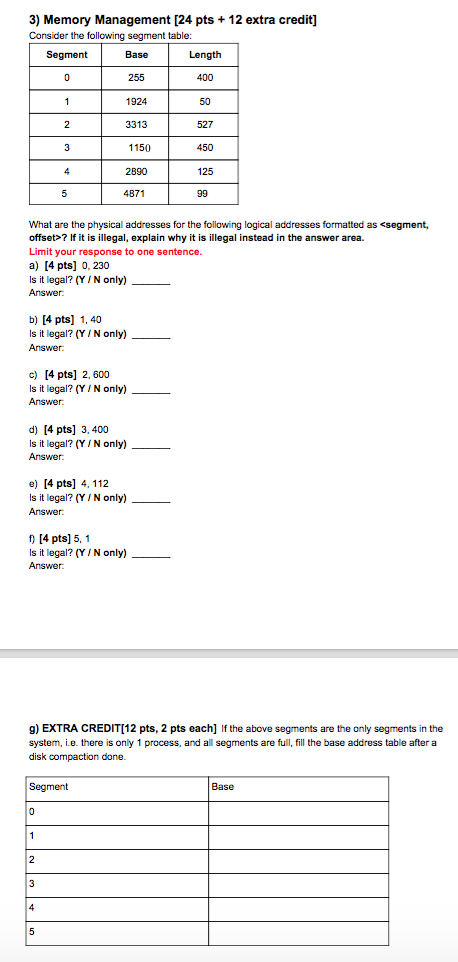3) Memory Management [24 pts 12 extra credit] Consider the following segment table: Base 255 400 1924 50 3313 1150 2B90 125 4871 What are the physical addresses for the following logical addresses formatted as <segment, offset>? If it is illegal, explain why it is illegal instead in the answer area. Limit your response to one sentence. a) [4 pts] 0, 230 Is it legal? (Y IN only) b) [4 pts] 1, 40 Is it legal? (Y , N only)...

Need Online Homework Help?